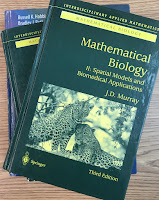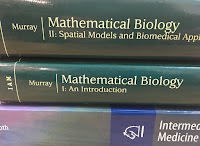## Friday, August 5, 2011

### Fisher-Kolmogorov equationMathematical Biology, by James Murray.
In the 4th edition of Intermediate Physics for Medicine and Biology, Russ Hobbie and I discuss many of the important partial differential equations of physics, such as Laplace’s equation, the diffusion equation, and the wave equation. One lesser-known PDE that we don’t discuss is the Fisher-Kolmogorov equation. However, our book supplies most of what you need to understand this equation.

In Section 2.10, we examine the logistic equation, an ordinary differential equation governing population growth,

du/dt = b u (1 − u) .

For u much less than one, the population grows exponentially with rate b. As u approaches one, the population levels off near a steady state value of u = 1. Our Eq. 2.28 gives an analytical solution to this nonlinear equation.

In Section 4.8, we drive the diffusion equation, which for one dimension is

du/dt = D d2u/dx2 .

This linear partial differential equation is one of the most famous in physics. It describes diffusion of particles, and also the flow of heat by conduction. D is the diffusion constant.

To get the Fisher-Kolmogorov equation, just put the logistic equation and the diffusion equation together:

du/dt = D d2u/dx2 + b u (1 u) .

The Fisher-Kolmogorov equation is an example of a “reaction-diffusion equation.” Russ and I discuss a similar reaction-diffusion equation in Homework Problem 24 of Chapter 4, when modeling intracellular calcium waves. The only difference is that we use a slightly more complicated reaction term rather than the logistic equation.Mathematical Biology, by James Murray.
In his book Mathematical Biology, James Murray discusses the Fisher-Kolmogorov equation in detail. He states
The classic simplest case of a nonlinear reaction diffusion equation … is [The Fisher-Kolmogorov equation]… It was suggested by Fisher (1937) as a deterministic version of a stochastic model for the spatial spread of a favoured gene in a population. It is also the natural extension of the logistic growth population model discussed in Chapter 11 when the population disperses via linear diffusion. This equation and its travelling wave solutions have been widely studied, as has been the more general form with an appropriate class of functions f(u) replacing ku(1u). The seminal and now classical paper is that by Kolmogoroff et al. (1937)…. We discuss this model equation in the following section in some detail, not because in itself it has such wide applicability but because it is the prototype equation which admits travelling wavefront solutions. It is also a convenient equation from which to develop many of the standard techniques for analyzing single-species models with diffusive dispersal.”
The Fisher-Kolmogorov equation was derived independently by Ronald Fisher (1890–1962), an English biologist, and Andrey Kolmogorov (1903–1987), a Russian mathematician. The key original papers are
Fisher, R. A. (1937) “The Wave of Advance of Advantageous Genes.” Annals of Eugenics, Volume 7, Pages 353–369.

Kolmogoroff, A., I. Petrovsky, and N. Piscounoff (1937) “Etude de l’equation de la diffusion avec croissance de la quantite de matiere et son application a un probleme biologique.” Moscow University Mathematics Bulletin, Volume 1, Pages 1–25.